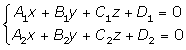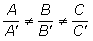Learn from home

The teachersGiven two planes:Form a system with the equations of the planes and calculate the ranks.r = rank of the coefficient matrix.

r'= rank of the augmented matrix.

The relationship between the two planes can be described as follows:

Positionrr'
Intersecting22Parallel12Coincident11State the relationship between the planes.

1.For intersecting planes, the intersection is a line.2.Parallel planes.

3.Coincident planes.

Did you like the article?(1 votes, average: 5.00 out of 5)Loading...

Emma

I am passionate about travelling and currently live and work in Paris. I like to spend my time reading, gardening, running, learning languages and exploring new places.

Did you like
this resource?

Bravo!